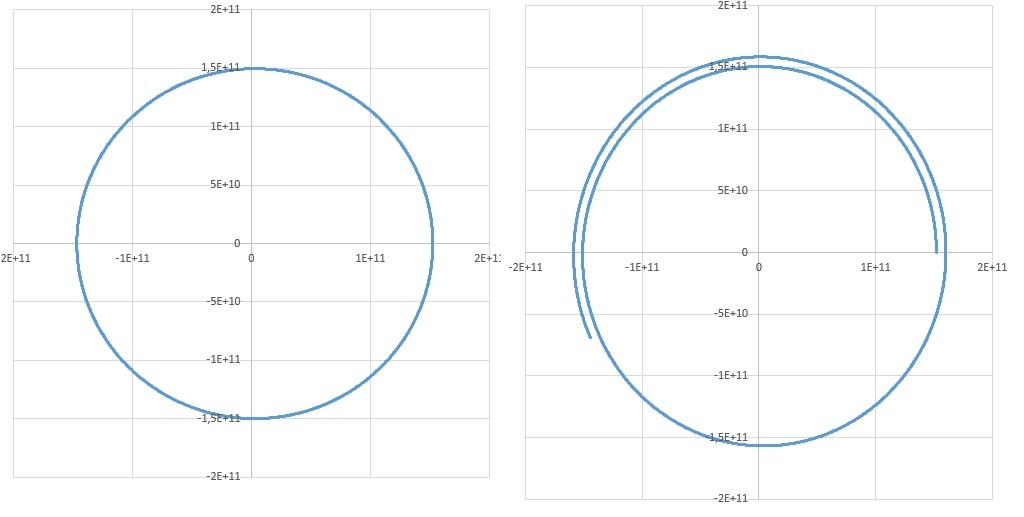# The order of operations

Up a level : Differential Equations
Previous page : The Earth orbit and Euler’s Step method
Next page : Homogenous differential equations - a more general typeSo let us test both ways. In the figure below the left one is calculating both the position and the velocity using the values from the previous round of calculations, i.e. the positions are calculated first, before the velocities have been updated, then the velocities are calculated. In the right figure is was calculated as described in the previous page, i.e. the velocities are updated first, then the new velocities are used in the calculations of the new positions.As one can see, the leftmost figure is way more accurate.  This is done in this (Sun Earth) Excel file with a Δt=1/4 of a day=21600 s. The left figure correspond to the Excel page 1, and the right to page 2.

According to the better variant of the simulation the Perihelion/closest distance to the Sun would be 147187048 km and in reality it is 14709500 km, so we are off with just one part in 1600. Not too bad given the step size.

So why the difference? Basically, in the good algorithm,  the errors interacting in a cancelling way.  The Euler step method have a tendency to overshoot, since it follows the tangent line. By using an earlier value of the velocity the algorithm will undershoot, and these two errors cancel in a surprisingly helpful way.

(The same trick is used in the simple harmonic motion simulation. )

We could still improve upon the above by adding more steps, also let the sun be influenced by the gravity, so that the sun will “wobble” in its orbit, and finally by using a better step method.Up a level : Differential Equations
Previous page : The Earth orbit and Euler’s Step method
Next page : Homogenous differential equations - a more general typeLast modified: Sep 21, 2019 @ 18:45